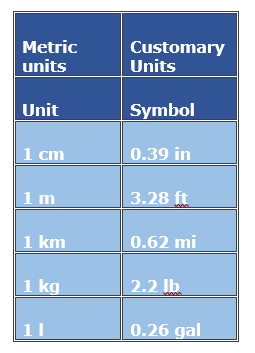# Converting between Metric and U.S. Customary Unit Systems

The conversion between Metric Units and Customary Units can be done using the table below. The units relate to measurement of length, weight and capacity.

In this lesson, we solve problems on conversion between metric units and US customary units for length, weight, capacity and time.A wooden stick is 29 inches long? How long is the stick in centimeters? Use the following conversion: 1 in = 2.54 cm.

### Solution

Step 1:

1 in = 2.54 cm

Step 2:

29 inches = 29 × 2.54 cm = 73.66 cm

So, the length of the stick in centimeters is 73.66 cm

The weight of a sack of potatoes is 27 kg. How much would the sack of potatoes weigh in pounds. Use the following conversion: 1 kg = 2.2 lb

### Solution

Step 1:

Given 1 kilogram = 2.2 pounds

Step 2:

27 kilograms = 27 kg/1 × 2.2 pounds/ 1 kg = 59.4 pounds

So, 27 kilograms = 59.4 pounds

The capacity of a can of syrup is 14 gallons. What is the capacity of the can of syrup in liters? Use the conversion: 1 gallon = 3.79 liters

### Solution

Step 1:

1 gallon = 3.79 liters

Step 2:

14 gallons = 14 gallons/1 × 3.79 liters/ 1 gallon = 53.06 liters

So, the capacity of the can of syrup in liters = 53.06 liters